Inequalities on a Number Line

# Inequalities on a Number Line

GCSE(F), GCSE(H),

Inequalities are used when a number is less than or greater than another value and will use one of the inequality signs:

< less than

≤ less than or equal to;

> greater than;

≥ greater than or equal to.

When used algebraically, the inequality will refer to a number, or range of numbers, which are either greater (or equal to), or less than (or equal to) a fixed value.

This can be shown on a number line using lines and circles. If a circle is filled, then it is equal to or greater/less than the value. If the circle is not filled, then the value is not included. A line, with an arrow, shows the range of values. Three examples are shown below:

a) x < 3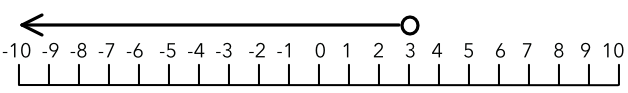b) x ≤ 3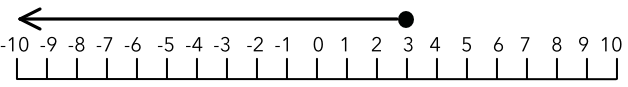c) x ≥ -2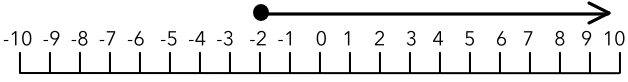Two inequalities can be applied to a value at the same time. On a number line, this would be represented by a line with a circle at each end. Show, on a number line, the values of x that satisfy x > -3 and x < 5: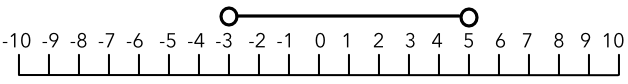## Examples

1. Show, on a number line, the inequality x > 6.

Answer: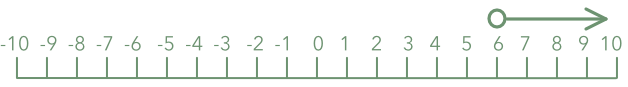The greater than sign indicates that the values must be greater than 6, therefore the circle must not be filled. The arrow indicates that all numbers greater than 6 are included.

2. What inequality is shown by the number line, below?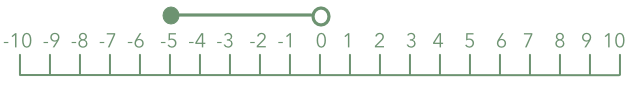Answer: -5 ≤ x < 0

x is greater than or equal to -5; and x is less than 0. Note that the filled circle indicates equal to or greater than; and the circle that is not filled indicates less than.